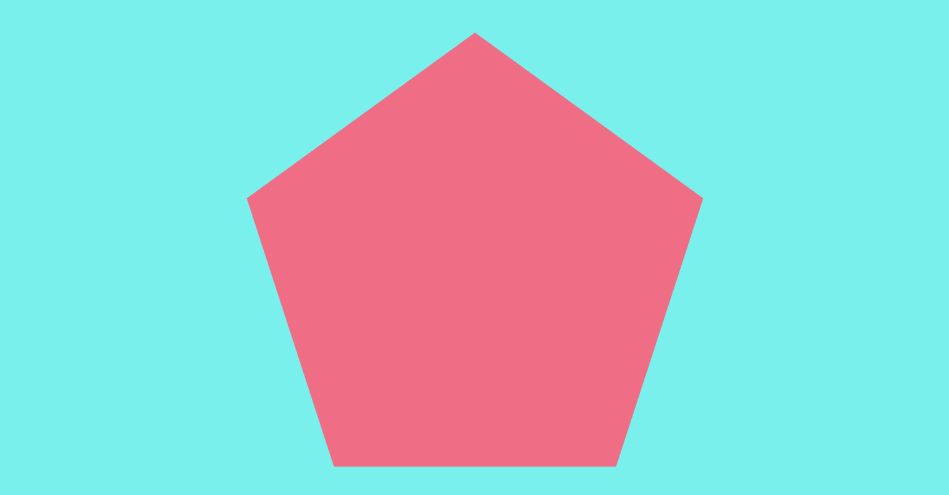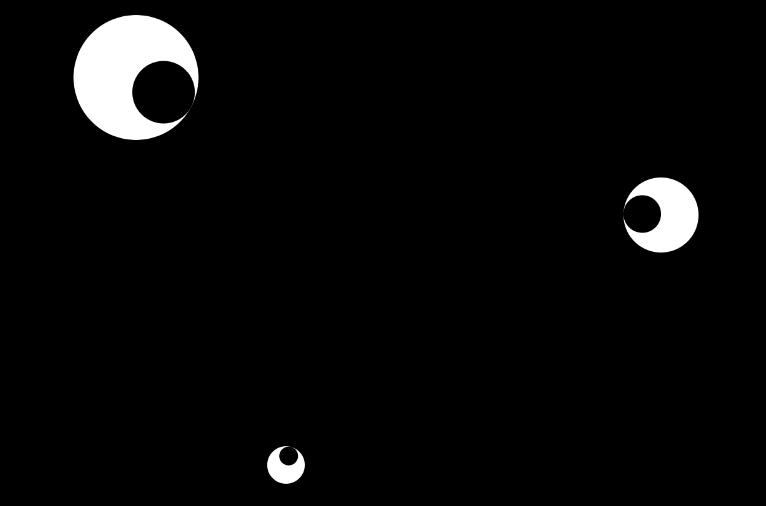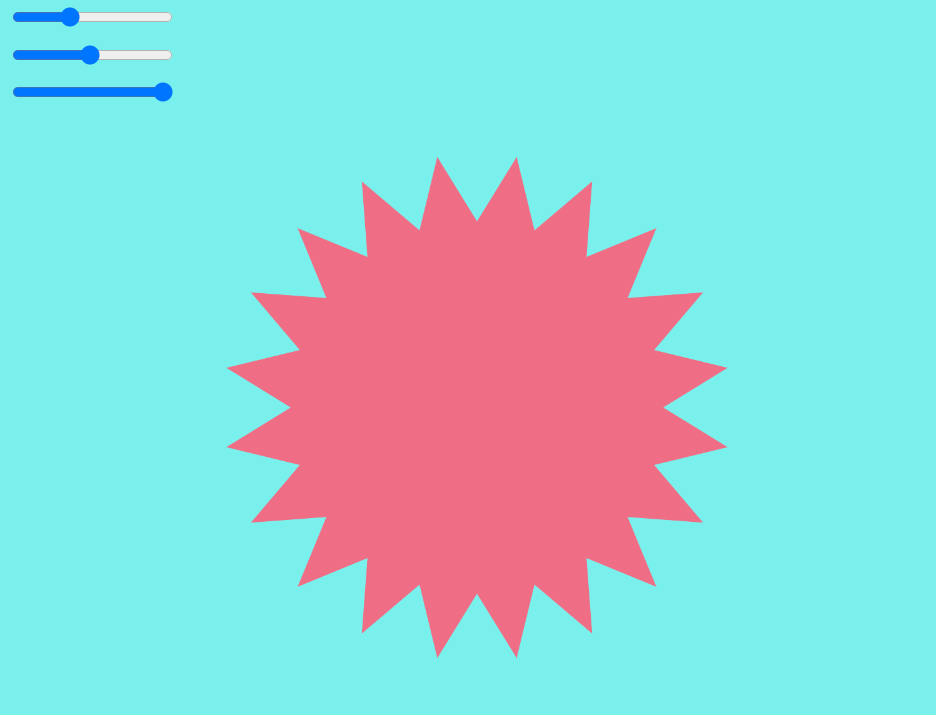# p5: Functions (optional)Go through the functions tutorial

Recording of the class lesson here

Exercise 1Part 1: Starting from this sketch, make a function to draw eyes at specified positions.

Part 2: add a parameter that specifies the size (diameter or scale) of the eye you draw so it can be like this one

Exercise 2Starting from this sketch, make a function to draw stars based on the parameters specified with the sliders.
It should function like this one.

Exercise 3

Make a function that calculates and returns the average of a series of numbers passed as an array:

```let myNumbers = [3, 20, 45, 1, -20];
let a = average(myNumbers);
print("The average is "+a);```

The average is calculated by adding the values together and then dividing the total by the number of values (the length of the array).